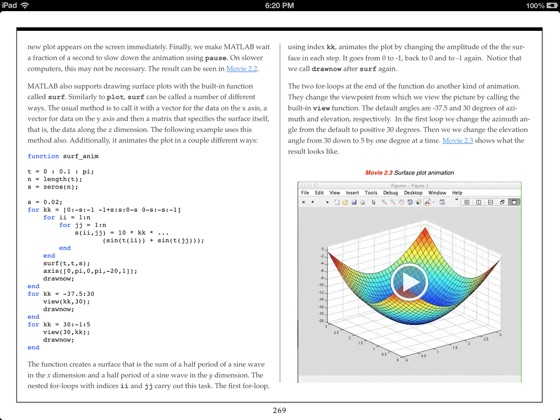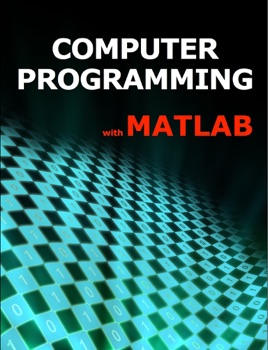• 9,99 €

## Publisher Description

The primary purpose of this book is to teach computer programming to those with little to no previous experience. It uses the programming system and language called MATLAB to do so because MATLAB is easy to learn and, at the same time, is an extremely versatile and useful programming language and programming environment. MATLAB is a special-purpose language that is an excellent choice for writing moderate-size programs that solve problems involving the manipulation of numbers. The design of the language makes it possible to write a powerful program in a few lines. The problems may be relatively complex, while the MATLAB programs that solve them are relatively simple: relative, that is, to the equivalent program written in a general-purpose language, such as C++ or Java. As a result, MATLAB is being used in a wide variety of domains from the natural sciences through all disciplines of engineering to finance and beyond, and it is heavily used in industry. Hence, a solid background in MATLAB is an indispensable skill in today’s job market.

Nevertheless, this book is not merely a reference manual for MATLAB or a MATLAB tutorial. It is an introductory programming textbook that happens to use MATLAB to illustrate general concepts in computer science and programming. As a side effect, the reader will gain a solid foundation in MATLAB. This book is a good fit for an introductory college-level course in computer programming for engineering and science students. In fact, it is being used as the textbook for such a course at Vanderbilt University. It serves the dual purpose of teaching computer programming and providing a background in MATLAB, which is used in higher-level courses in many majors. This book is also suitable to teach programming to high school students. The material assumes no background in mathematics that is not part of standard high school curricula, and MATLAB is much more accessible as an introduction to programming to the average student than Java, C, C++, or other general-purpose languages.

•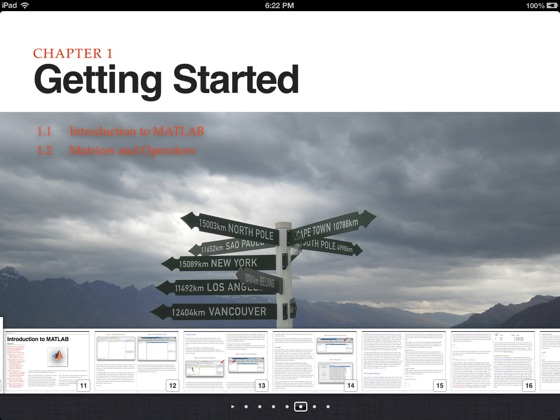•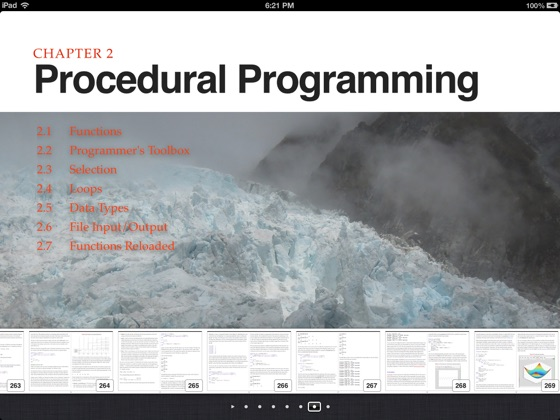•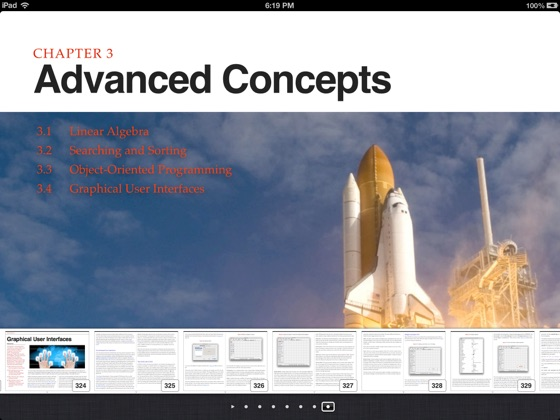••